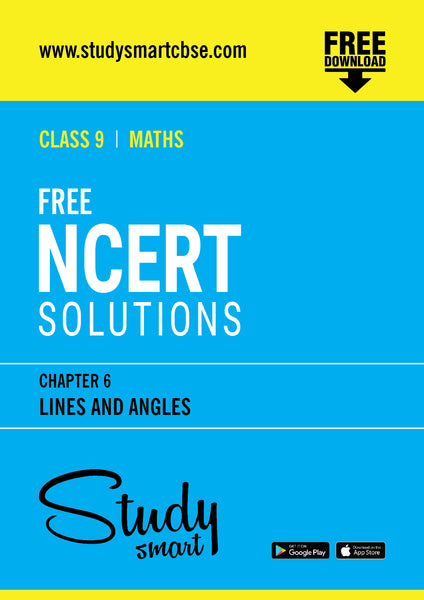# Free NCERT Solutions Class 9th Maths Chapter 6 Lines and Angles

This will be sent as a link to your registered email id

#### Description:

Download Free NCERT Solutions Class 9th Maths Chapter 6 Lines and Angles

1. (Motivate) If a ray stands on a line, then the sum of the two adjacent angles so formed is 180° and the converse.

2. (Prove) If two lines intersect, vertically opposite angles are equal.

3. (Motivate) Results on corresponding angles, alternate angles, interior angles when a transversal intersects two parallel lines.

4. (Motivate) Lines which are parallel to a given line are parallel.

5. (Prove) The sum of the angles of a triangle is 180° .

6. (Motivate) If a side of a triangle is produced, the exterior angle so formed is equal to the sum of the two interior opposite angles.